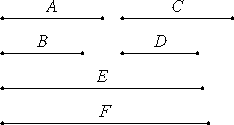# Proposition 26

If two numbers are relatively prime to two numbers, both to each, then their products are also relatively prime.

Let the two numbers A and B be relatively prime to the two numbers C and D, both to each, and let A multiplied by B make E, and let C multiplied by D make F.

I say that E and F are relatively prime.VII.24

Since each of the numbers A and B is relatively prime to C, therefore the product of A and B is also relatively prime to C. But the product of A and B is E, therefore E and C are relatively prime. For the same reason E and D are also relatively prime. Therefore each of the numbers C and D is relatively prime to E.

VII.24

Therefore the product of C and D is also relatively prime to E. But the product of C and D is F. Therefore E and F are relatively prime.

Therefore, if two numbers are relatively prime to two numbers, both to each, then their products are also relatively prime.

Q.E.D.

## Guide

This proposition says that if a and b are each relatively prime to both c and d, then ab is relatively prime to cd.

For example, 45 and 75 are each relatively prime to 26 and 77, so 45·75, which is 3375, and 26·77, which is 1144, are relatively prime.

The proof of this proposition uses proposition VII.24 twice. If a and b are both relatively prime to both c and d, then so is their product ab. Now since c and d are both relatively prime to ab, therefore so is their product cd.

This proposition is used in the proof of the next one.